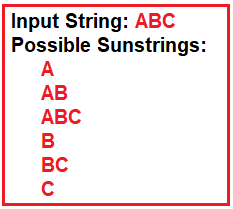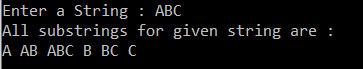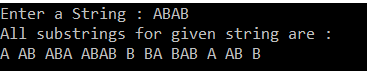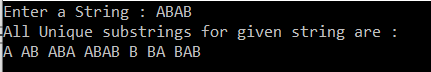# How to Find All Substrings of a Given String in C#

## How to Find All Substrings of a Given String in C#

In this article, I am going to discuss How to Find All Substrings of a Given String in C# with some examples. Please read our previous article where we discussed how to remove duplicate characters from a string in C# with some examples. As part of this article, we are going to implement the above program with the following ways.

1. Without and with using Built-in Methods to Find all Possible Substrings of a Given String.
2. How to find only the unique substrings of a given string in C#.
3. Using Linq to Find ALL possible substring as well as Unique substring of a given string.
##### Program Description:

Here, we will take the input as a string from the console and then need to print all the possible substrings of that string. The substring of a given string is nothing but the characters or the group of characters that are present within the string. To understand this better please have a look at the following diagram which shows the string and its possible substrings.##### Algorithm to find all possible substring of a given string:

Step1: Define a string.
Step2: The first loop (i.e. the outer loop) will keep the first character of the substring.
Step3: The second loop (i.e. the inner loop) will build the substring by adding one character in each iteration till the end of the string is reached.
For Example, if the given String is “ABCD”
Then the first loop will hold the position of A, then B then C and finally D
The second loop will be substring the string into
For i=1: A, AB, ABC, then ABCD for the last iteration
For i=2: B, BC and then BCD
For i=3: C and then CD
For i=4: D
Step4: Print the substring

##### Using a Simple Approach:

In the following example, we used two loops. The outer loop is used to maintain the relative position of the first character and the inner loop is used to create all possible substrings one by one and print them on the console.

```using System;
using System.Text;

namespace LogicalPrograms
{
class Program
{
static void Main(string[] args)
{
Console.Write("Enter a String : ");

Console.WriteLine("All substrings for given string are : ");

for (int i = 0; i < inputString.Length; ++i)
{
StringBuilder subString = new StringBuilder(inputString.Length - i);
for (int j = i; j < inputString.Length; ++j)
{
subString.Append(inputString[j]);
Console.Write(subString + " ");
}
}

}
}
}
```

Output:##### Using Substring method:

In the following example, we use the built-in substring method to create the string. The Substring (i, len) creates a substring of length ‘len’ starting from index i in the given string.

```using System;
namespace LogicalPrograms
{
class Program
{
static void Main(string[] args)
{
Console.Write("Enter a String : ");

int len = inputString.Length;
Console.WriteLine("All substrings for given string are : ");

//This loop maintains the starting character
for (int i = 0; i < len; i++)
{
//This loop adds the next character every iteration for the substring and then print
for (int j = 0; j < len - i; j++)
{
Console.Write (inputString.Substring(i, j + 1) + " ");
}
}

}
}
}
```

Output:As you can see in the above output, it prints all the possible substrings of a given string. Further, if you observe it is not maintaining the uniqueness of the strings. That is some substrings (such as A, AB, B) are appear multiple times.

##### Finding Unique Substrings of a Given String in C#:

In the following example, we are storing all the possible substrings into an array. Then use the Linq Distinct method to get the distinct values only.

```using System.Linq;
using System;

namespace LogicalPrograms
{
class Program
{
static void Main(string[] args)
{
Console.Write("Enter a String : ");

int len = inputString.Length;
int temp = 0;

//Total possible substrings for string of size n is n*(n+1)/2
String[] SubstringArray = new String[len * (len + 1) / 2];

//This loop maintains the starting character
for (int i = 0; i < len; i++)
{
//This loop adds the next character every iteration for the substring
//and then store into the array
for (int j = 0; j < len - i; j++)
{
SubstringArray[temp] = inputString.Substring(i, j + 1);
temp++;
}
}

//Get the distinct array
SubstringArray = SubstringArray.Distinct().ToArray();

//Print the array
Console.WriteLine("All Unique substrings for given string are : ");
for (int i = 0; i < SubstringArray.Length; i++)
{
Console.Write(SubstringArray[i] + " ");
}

}
}
}
```

Output:Note: All the possible substrings for a string will be n*(n + 1)/2. So, here we are creating the string array with the size n*(n+1)/2.

##### Using LINQ to Find All and Unique Substrings of a Given String in C#:

In the following example, we show you how to use LINQ query to find all the possible substrings as well as unique strings of a given string.

```using System.Linq;
using System;

namespace LogicalPrograms
{
class Program
{
static void Main(string[] args)
{
Console.Write("Enter a String : ");

var Substrings =
from i in Enumerable.Range(0, inputString.Length)
from j in Enumerable.Range(0, inputString.Length - i + 1)
where j >= 1
select inputString.Substring(i, j);

//Print the array
Console.WriteLine();
Console.WriteLine("All substrings for given string are : ");
foreach (string substring in Substrings)
{
Console.Write(substring + " ");
}

Console.WriteLine();
Console.WriteLine();
Console.WriteLine("All Unique substrings for given string are : ");
foreach (string substring in Substrings.Distinct())
{
Console.Write(substring + " ");
}